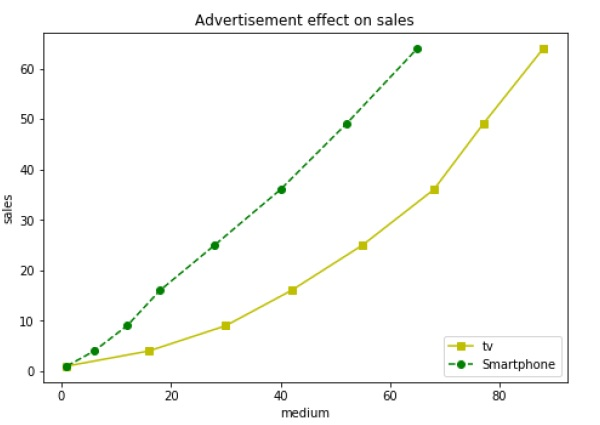# Matplotlib - Axes Class

Axes object is the region of the image with the data space. A given figure can contain many Axes, but a given Axes object can only be in one Figure. The Axes contains two (or three in the case of 3D) Axis objects. The Axes class and its member functions are the primary entry point to working with the OO interface.

Axes object is added to figure by calling the add_axes() method. It returns the axes object and adds an axes at position rect [left, bottom, width, height] where all quantities are in fractions of figure width and height.

## Parameter

Following is the parameter for the Axes class −

• rect − A 4-length sequence of [left, bottom, width, height] quantities.

```ax=fig.add_axes([0,0,1,1])
```

The following member functions of axes class add different elements to plot −

## Legend

The legend() method of axes class adds a legend to the plot figure. It takes three parameters −

```ax.legend(handles, labels, loc)
```

Where labels is a sequence of strings and handles a sequence of Line2D or Patch instances. loc can be a string or an integer specifying the legend location.

Location string Location code
Best 0
upper right 1
upper left 2
lower left 3
lower right 4
Right 5
Center left 6
Center right 7
lower center 8
upper center 9
Center 10

## axes.plot()

This is the basic method of axes class that plots values of one array versus another as lines or markers. The plot() method can have an optional format string argument to specify color, style and size of line and marker.

Character Color
‘b’ Blue
‘g’ Green
‘r’ Red
‘b’ Blue
‘c’ Cyan
‘m’ Magenta
‘y’ Yellow
‘k’ Black
‘b’ Blue
‘w’ White

## Marker codes

Character Description
‘.’ Point marker
‘o’ Circle marker
‘x’ X marker
‘D’ Diamond marker
‘H’ Hexagon marker
‘s’ Square marker
‘+’ Plus marker

## Line styles

Character Description
‘-‘ Solid line
‘—‘ Dashed line
‘-.’ Dash-dot line
‘:’ Dotted line
‘H’ Hexagon marker

Following example shows the advertisement expenses and sales figures of TV and smartphone in the form of line plots. Line representing TV is a solid line with yellow colour and square markers whereas smartphone line is a dashed line with green colour and circle marker.

```import matplotlib.pyplot as plt
y = [1, 4, 9, 16, 25,36,49, 64]
x1 = [1, 16, 30, 42,55, 68, 77,88]
x2 = [1,6,12,18,28, 40, 52, 65]
fig = plt.figure()
l1 = ax.plot(x1,y,'ys-') # solid line with yellow colour and square marker
l2 = ax.plot(x2,y,'go--') # dash line with green colour and circle marker
ax.legend(labels = ('tv', 'Smartphone'), loc = 'lower right') # legend placed at lower right
ax.set_xlabel('medium')
ax.set_ylabel('sales')
plt.show()
```

When the above line of code is executed, it produces the following plot −